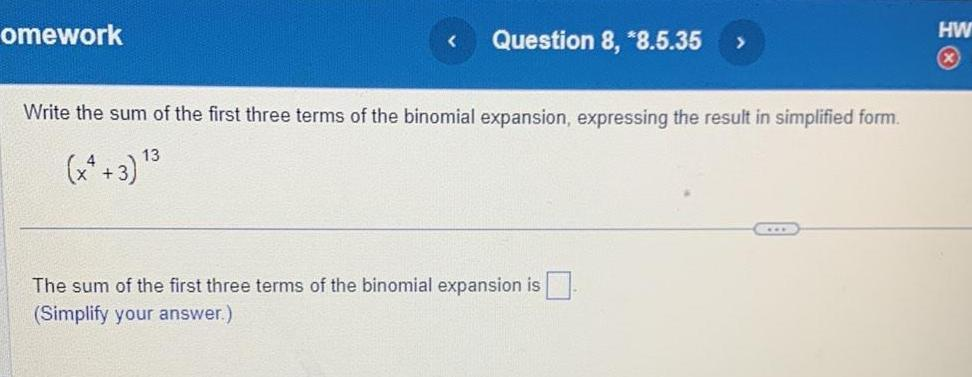Question:

# omework Question 8 8 5 35 Write the sum of the first three

Last updated: 11/19/2023omework Question 8 8 5 35 Write the sum of the first three terms of the binomial expansion expressing the result in simplified form 13 The sum of the first three terms of the binomial expansion is Simplify your answer HW x